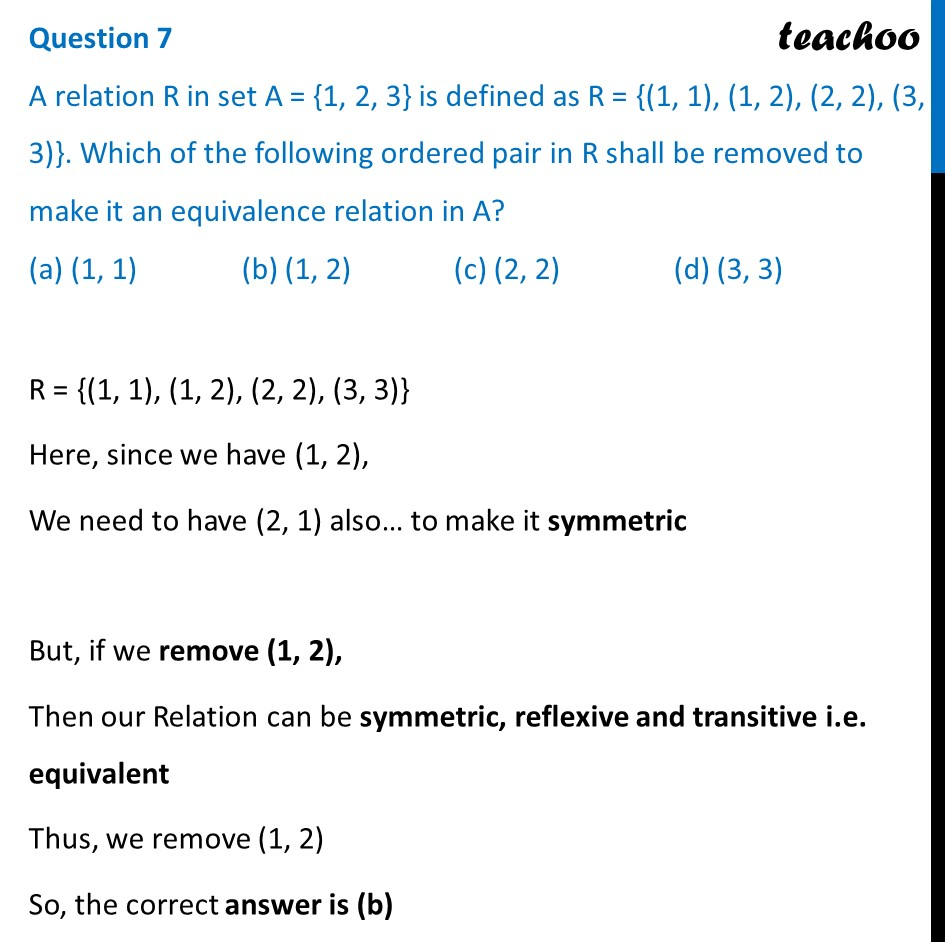CBSE Class 12 Sample Paper for 2022 Boards (MCQ Based - for Term 1)

Class 12
Solutions of Sample Papers and Past Year Papers - for Class 12 Boards

## (a) (1, 1)   (b) (1, 2)  (c) (2, 2)  (d) (3, 3)

This question is inspired from - Question 2 - CBSE Class 12 Sample Paper for 2021 BoardsLearn in your speed, with individual attention - Teachoo Maths 1-on-1 Class

### Transcript

Question 7 A relation R in set A = {1, 2, 3} is defined as R = {(1, 1), (1, 2), (2, 2), (3, 3)}. Which of the following ordered pair in R shall be removed to make it an equivalence relation in A? (a) (1, 1) (b) (1, 2) (c) (2, 2) (d) (3, 3) R = {(1, 1), (1, 2), (2, 2), (3, 3)} Here, since we have (1, 2), We need to have (2, 1) also… to make it symmetric But, if we remove (1, 2), Then our Relation can be symmetric, reflexive and transitive i.e. equivalent Thus, we remove (1, 2) So, the correct answer is (b)